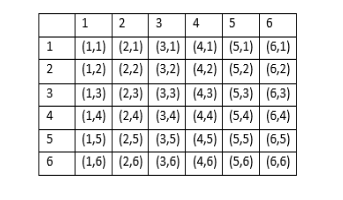QuestionAnswers

# A and B throw a pair of dice alternatively. A wins the game if he gets a total of 9 and B wins if he gets a total of 7. If A starts the game, find the probability of winning the game by B.

Hint: Start by finding the probability of getting the sum as 9, 7 and not getting the sum as 9 and 7. Then focus on the point that the possibilities that A wins the game, is A wins the game in the first throw; A don’t win in the first throw and B also doesn't get the sum of 7 in his chance then A gets the sum 9 and so on. Representing this mathematically would give an infinite GP and the sum of an infinite GP is given by $\dfrac{a}{1-r}$ , where a is the first term and r is the common ratio. Solve the GP and get the answer.

Before moving to the question, let us talk about probability.
Probability in simple words is the possibility of an event to occur.
Probability can be mathematically defined as $=\dfrac{\text{number of favourable outcomes}}{\text{total number of outcomes}}$ .
Start by drawing the table of all the possible outcomes. There would be a total of 36 outcomes, as 6 options for each dice and both dices are thrown together. The total number of outcomes for each of the cases is 36.Let us start by finding the probability of getting a sum of 9. The favourable outcomes, i.e, the sum as 9 are (4,5), (6,3), (3,6), (5,4). So, the number of favourable outcomes are 4.
$\text{P}\left( \text{sum equals 9} \right)=\dfrac{4}{36}=\dfrac{1}{9}$
So, the probability of not getting the sum 9 is:
$\overline{\text{P}\left( \text{sum equals 9} \right)}=1-\dfrac{1}{9}=\dfrac{8}{9}$
Now, let us find the probability of getting a sum of 7. The favourable outcomes, i.e, the sum as 7 are (4,3), (5,2), (3,4), (2,5), (1,6), (6,1). So, the number of favourable outcomes are 6.
$\text{P}\left( \text{sum equals 7} \right)=\dfrac{6}{36}=\dfrac{1}{6}$
So, the probability of not getting the sum 7 is:
$\overline{\text{P}\left( \text{sum equals 7} \right)}=1-\dfrac{1}{6}=\dfrac{5}{6}$
Now, let us find the probability of A winning the game. So, the possibilities that A wins the game, is A wins the game in the first throw; A doesn't win in the first throw and B also doesn't get the sum of 7 in his chance, then A gets the sum 9 and so on.
$P\left( \text{A wins} \right)=\dfrac{1}{9}+\dfrac{8}{9}\times \dfrac{5}{6}\times \dfrac{1}{9}+\dfrac{8}{9}\times \dfrac{5}{6}\times \dfrac{8}{9}\times \dfrac{5}{6}\times \dfrac{1}{9}.................$
So, if you observe the series, it is an infinite GP with first term as $\dfrac{1}{9}$ and common ratio as $\dfrac{8}{9}\times \dfrac{5}{6}=\dfrac{20}{27}$ . We know that the sum of an infinite GP is given by $\dfrac{a}{1-r}$ , where a is the first term and r is the common ratio.
$P\left( \text{A wins} \right)=\dfrac{\dfrac{1}{9}}{1-\dfrac{20}{27}}=\dfrac{\dfrac{1}{9}}{\dfrac{7}{27}}=\dfrac{3}{7}$
Hence, the probability that A wins the game is $\dfrac{3}{7}$ and the probability that B wins is 1 minus the probability that A wins, i.e., $1-\dfrac{3}{7}=\dfrac{4}{7}$ .

Note: Remember that the formula for the sum of the infinite GP is only valid if the common ratio is less than 1, which was the case for the above question. Also, for finding the probability of B winning you could have used the same method as A but that would be lengthier and we know that there are only two participants, so if A doesn’t win B will definitely win, so the above used method is better.